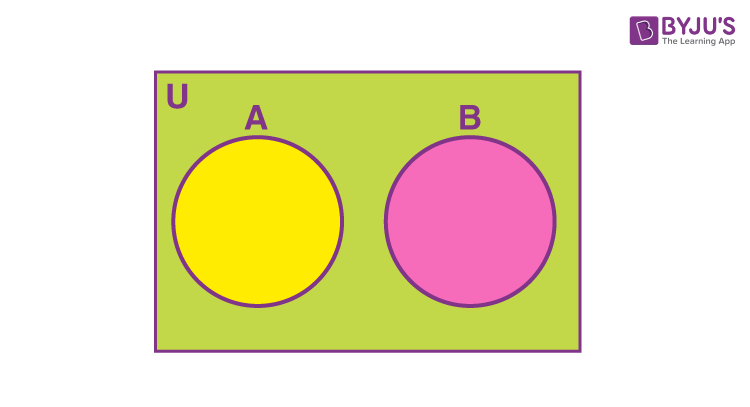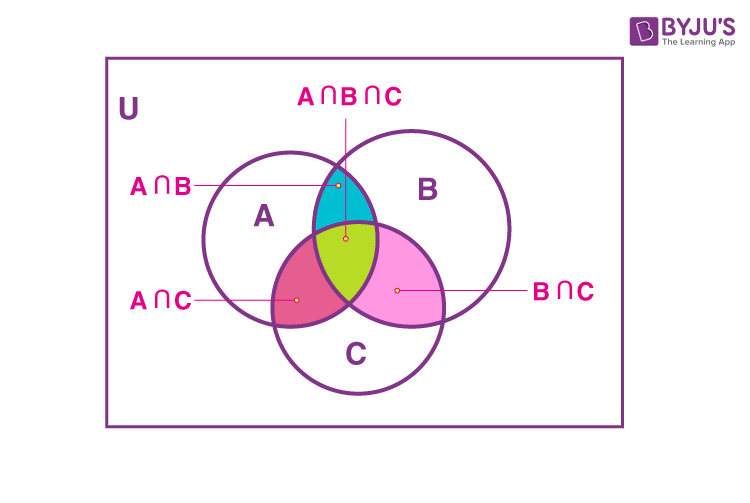# Formula Based On Cardinality Of Sets

The number of elements in a set is called the cardinality of a set. For example, let A = {h, i, j, k, l}. Then the cardinality of set A is denoted by n(A). There are 5 elements in set A. So n(A) = 5. If two or more sets are combined using the operations on sets, using the formula based on cardinality of sets, we can calculate the cardinality.

Formulas based on cardinality of sets are given below.

1. Let A and B be finite sets and (A⋂B) ≠ φ, then

n(A⋃B) = n(A) + n(B) – n(A⋂B). ⋃ denotes union and ⋂ denotes intersection.

To find the cardinality of A⋃B, we subtract the number of common elements of A and B from the sum of cardinality of A and B.

2. If the sets A and B are disjoint sets (A and B do not have any common elements), n(A⋂B) = 0

n(A⋃B) = n(A) + n(B). The venn diagram given below gives a clear idea of this formula.3 . Let A, B and C be finite sets and (A⋂B⋂C) ≠ φ, then

n(A⋃B⋃C) = n(A) + n(B) + n(C) – n(A⋂B) – n(B⋂C) – n(C⋂A) + n(A⋂B⋂C)4. If A, B and C are disjoint sets, then

n(A⋂B) = 0, n(B⋂C) = 0, n(C⋂A) = 0, n(A⋂B⋂C) = 0

=> n(A⋃B⋃C) = n(A) + n(B) + n(C)

### Solved Examples

Example 1:

In a college there are 25 teachers who teach physics or mathematics. Of these, 15 teach physics and 6 teach both physics and mathematics. How many teach mathematics?

a. 15

b. 16

c. 10

d. None of these

Solution:

Let A denotes the number of teachers who teach physics and B denotes the number of teachers who teach mathematics.

Total number of teachers who teach physics or maths, n(A⋃B) = 25

Number of physics teachers, n(A) = 15

Number of teachers who teach both mathematics and physics, n(A⋂B) = 6

n(A⋃B) = n(A) + n(B) – n(A⋂B)

=> 25 = 15 + n(B) – 6

=> n(B) = 16

Hence option b is the answer.

Example 2:

In a group of 200 children, it was found that 120 children like cricket, 90 like tennis and 70 football, 40 like cricket and tennis, 30 like tennis and football, 50 like football and cricket and 20 like none of these games. Find the number of children who like all three games.

a. 15

b. 16

c. 20

d. None of these

Solution:

Let cricket = C, Tennis = T, Football = F

Given n(C) = 120

n(T) = 90

n(F) = 70

n(C⋂T) = 40

n(T⋂F) = 30

n(F⋂C) = 50

Given 20 children like none of the games.

n(C⋃T⋃F)’ = n(U) – n(C⋃T⋃F)

20 = 200 – n(C⋃T⋃F)

So n(C⋃T⋃F) = 180

n(C⋃T⋃F) = n(C) + n(T) + n(F) – n(C⋂T) – n(T⋂F) – n(F⋂C) + n(C⋂T⋂F)

180 = 120 + 90 + 70 – 40 – 30 – 50 + n(C⋂T⋂F)

=> n(C⋂T⋂F) = 180 – 160

= 20

So the number of children who like all the three games = 20

Hence, option c is the answer.

Related video